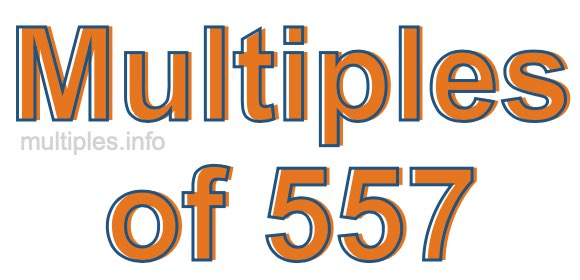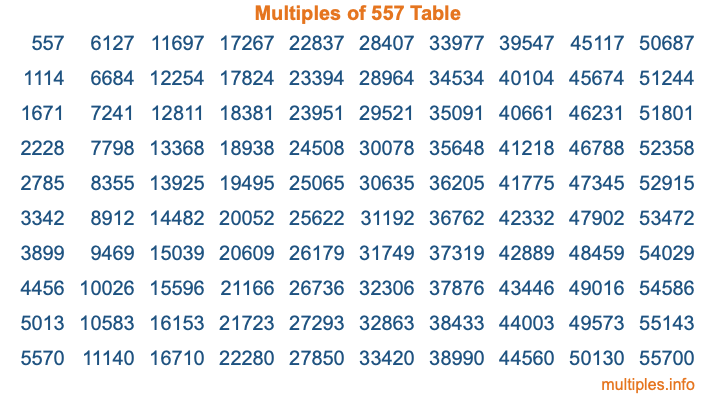Multiples of 557Welcome to the Multiples of 557 page. Here we will first teach you everything you will ever need to know about the multiples of 557, and then give you a study guide summary of everything we taught you to make sure you remember it all. Use this page to look up facts and learn information about the multiples of 557. This page will make you a multiples of five hundred fifty-seven expert!

Definition of Multiples of 557
Multiples of 557 are all the numbers that when divided by 557 equal an integer. Each of the multiples of 557 are called a multiple. A multiple of 557 is created by multiplying 557 by an integer.

Therefore, to create a list of multiples of 557, you start with 1 multiplied by 557, then 2 multiplied by 557, then 3 multiplied by 557, and so on for as long as you want. Thus, the list of the first five multiples of 557 is 557, 1114, 1671, 2228, and 2785. To see a larger list of multiples of 557, see the printable image of Multiples of 557 further down on this page. We also have a category where you can choose any nth multiple of 557.

Multiples of 557 Checker
The Multiples of 557 Checker below checks to see if any number of your choice is a multiple of 557. In other words, it checks to see if there is any number (integer) that when multiplied by 557 will equal your number. To do that, we divide your number by 557. If the the quotient is an integer, then your number is a multiple of 557.

Is  a multiple of 557?

Least Common Multiple of 557 and ...
A Least Common Multiple (LCM) is the lowest multiple that two or more numbers have in common. This is also called the smallest common multiple or lowest common multiple and is useful to know when you are adding our subtracting fractions. Enter one or more numbers below (557 is already entered) to find the LCM.

Check out our LCM Calculator if you need more details about the Least Common Multiple or if you need the LCM for different numbers for adding and subtraction fractions.

nth Multiple of 557
As we stated above, 557 is the first multiple of 557, 1114 is the second multiple of 557, 1671 is the third multiple of 557, and so on. Enter a number below to find the nth multiple of 557.

th multiple of 557

Multiples of 557 vs Factors of 557
557 is a multiple of 557 and a factor of 557, but that is where the similarities end. All postive multiples of 557 are 557 or greater than 557. All positive factors of 557 are 557 or less than 557.

Below is the beginning list of multiples of 557 and the factors of 557 so you can compare:

Multiples of 557: 557, 1114, 1671, 2228, 2785, etc.

Factors of 557: 1, 557

As you can see, the multiples of 557 are all the numbers that you can divide by 557 to get a whole number. The factors of 557, on the other hand, are all the whole numbers that you can multiply by another whole number to get 557.

It's also interesting to note that if a number (x) is a factor of 557, then 557 will also be a multiple of that number (x).

Multiples of 557 vs Divisors of 557
The divisors of 557 are all the integers that 557 can be divided by evenly. Below is a list of the divisors of 557.

Divisors of 557: 1, 557

The interesting thing to note here is that if you take any multiple of 557 and divide it by a divisor of 557, you will see that the quotient is an integer.

Multiples of 557 Table
Below is an image of the first 100 multiples of 557 in a table. The table is in chronological order, column by column. The first column has the first ten multiples of 557, the second column has the next ten multiples of 557, and so on.The Multiples of 557 Table is also referred to as the 557 Times Table or Times Table of 557. You are welcome to print out our table for your studies.

Negative Multiples of 557
Although not often discussed or needed in math, it is worth mentioning that you can make a list of negative multiples of 557 by multiplying 557 by -1, then by -2, then by -3, and so on, to get the following list of negative multiples of 557:

-557, -1114, -1671, -2228, -2785, etc.

Multiples of 557 Summary
Below is a summary of important Multiples of 557 facts that we have discussed on this page. To retain the knowledge on this page, we recommend that you read through the summary and explain to yourself or a study partner why they hold true.

There are an infinite number of multiples of 557.

A multiple of 557 divided by 557 will equal a whole number.

557 divided by a factor of 557 equals a divisor of 557.

The nth multiple of 557 is n times 557.

The largest factor of 557 is equal to the first positive multiple of 557.

557 is a multiple of every factor of 557.

557 is a multiple of 557.

A multiple of 557 divided by a divisor of 557 equals an integer.

557 divided by a divisor of 557 equals a factor of 557.

Any integer times 557 will equal a multiple of 557.

Multiples of a Number
Here you can get the multiples of another number, all with the same attention to detail as we did for multiples of 557 on this page.

Multiples of
Multiples of 558
Did you find our page about multiples of five hundred fifty-seven educational? Do you want more knowledge? Check out the multiples of the next number on our list!